# Overview

• Installation of webpage template
• Download the LTSpice files
• Simulation analysis
• Summary

# Webpage Installation

• Download Website Template (Zip file)
• Unzip the folder and accessing the directory InEl_P2019
• Copy the folder and rename to 2020_Group<A1<_V1_<Ahmed<
• Edititng and formatting with Adobe Dreamviewer

# Test for 4 Bit ADC and DAC

 - A 4 Bit ADC and DAC test is simulated in LTSPICE - The files are donloaded and LTSPICE are started. - The output file size can be limited by using .save dialog option. - Voltage source was added with a ramp from 0-1V with rise time of 655 µs. - The picture shows a ramp in the voltage and DAC ramp output voltage over 16 steps are observed. - Using the following .meas (measurement) statement to find the voltage levels. - 0.0625V is given for the code 000 at time 60 µs. No error in the voltage level is seen. It is an ideal ADC and DAC.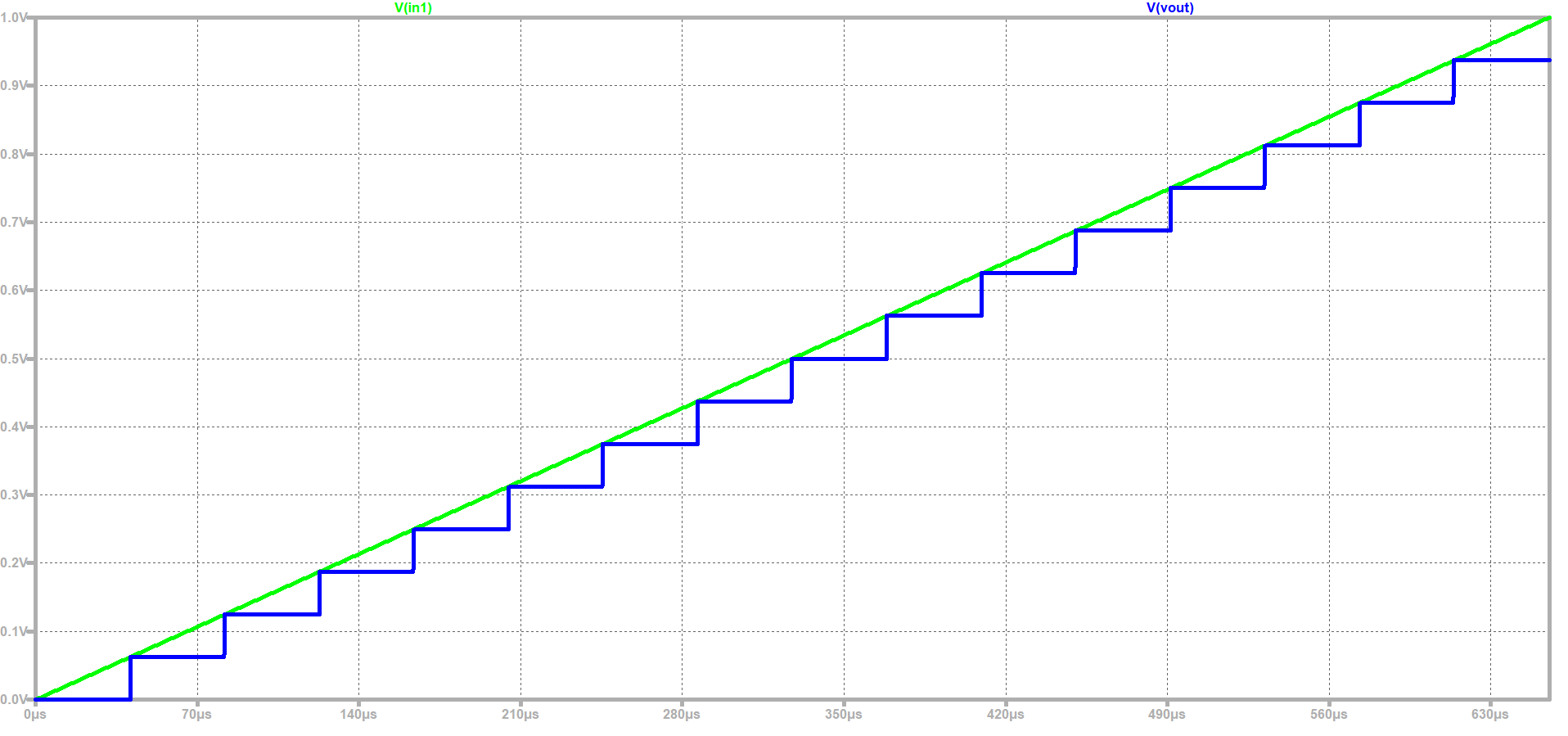# LTSPICE Schematics of 3Bit DAC

 Using the following .meas statement, the voltage levels are measured .meas tran V0000 FIND V(Vout) AT=0.9µs Similarly, At 1.95µs the voltage is shown as follows; V0001: V(Vout)=0.399999V at 1.95e-006 The given 3bitDAC circuit is totaly operated on transitor based switching. The spikes in the ouptup are becasue of the switching of the transistors .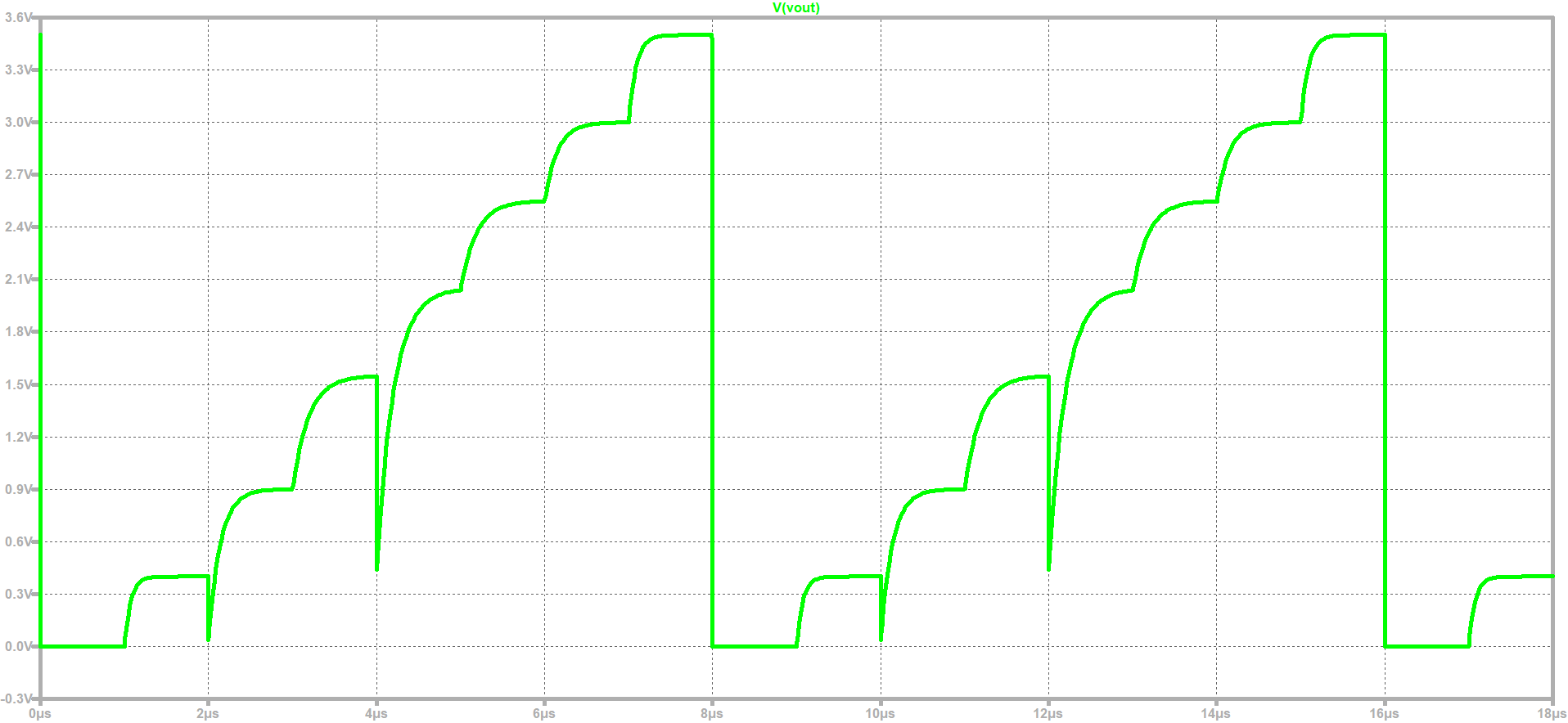# DNL and INL Analysis

• The DNL and INL values are calculated by using the following formulas
•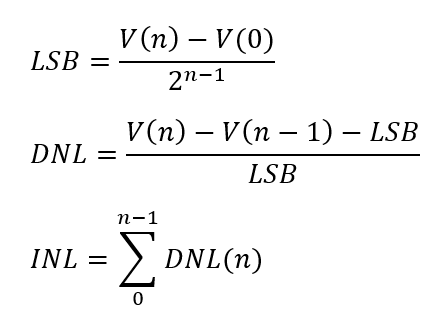• The graphs for the DNL, INL and Output voltage and Ideal Output voltage are shown below;
•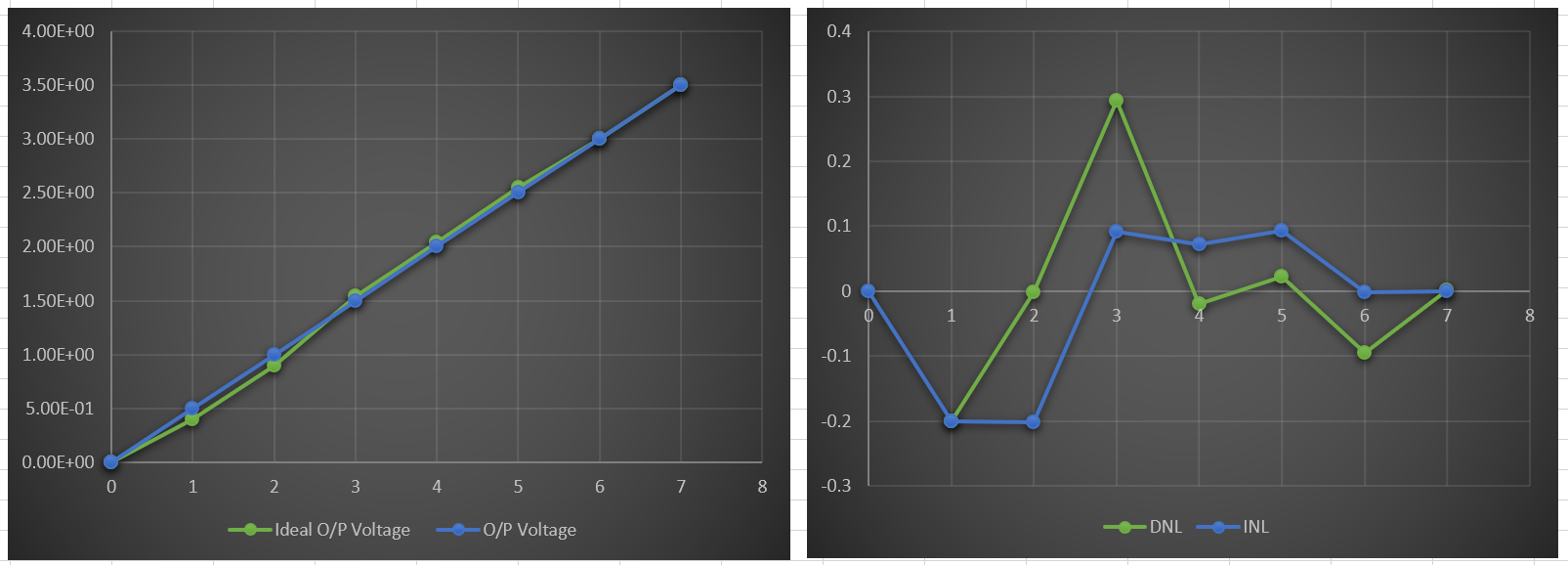• and their values are summarized in the following Table
•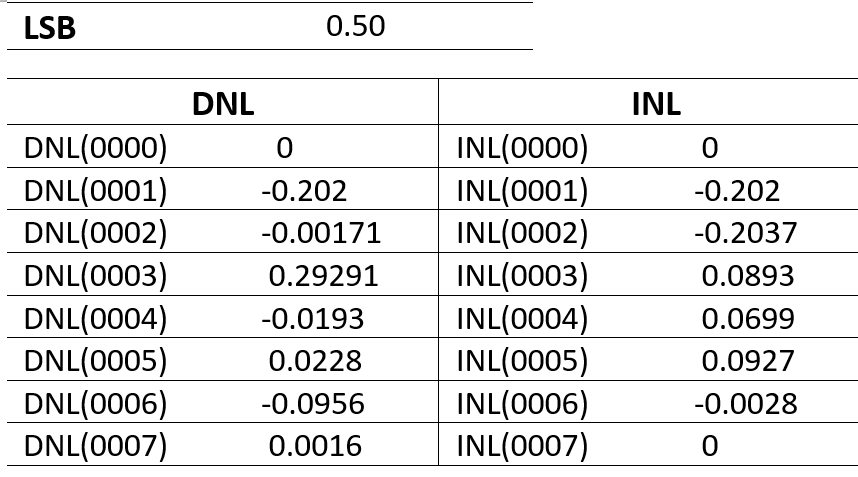# Summary

• A 4bit DAC and ADC is simulated in LT Spice and its output is analyzed
• No errors are observed in the ramp output voltage
• ALso a 3bit DAC is simulated on LTSpice and its output is analyzed
• Finally the complete lab tasks are reported in a webpage
• Note++ and Adobe Dreamviewer have been used to develop the webpage

# References

 Making of a Webreport , Vollrath